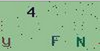• 注册

# php开发验证码（生成验证码）1.生成指定宽高的画布

2.准备好字好需要生成的字符串

3.每次执行，让背景填充随机的颜色（浅色系）

4.在画布上画上随机的干扰元素（随机点、随机线、随机弧形等均可，但不可过份影响用户的视觉）

5.写上4个文字

7.输出图像

8.销毁图像资源

`\$img = imagecreate(\$width, \$height);`

```function check_code(\$width = 100, \$height = 50) {
\$img = imagecreate(\$width, \$height);
}```

```   for (\$i = 0; \$i < \$num; \$i++) {
\$rand = mt_rand(0, 2);
switch (\$rand) {
case 0:
\$ascii = mt_rand(48, 57); //0-9
break;
case 1:
\$ascii = mt_rand(65, 90); //A-Z
break;
case 2:
\$ascii = mt_rand(97, 122); //a-z
break;
}
//chr()
\$string .= sprintf('%c', \$ascii);
}```

```//没有0,i,l,o
\$str = 'abcdefghijkmnpqrstuvwxyzABCDEFGHJKLMNPQRSTUVWXYZ123456789';
\$str = str_shuffle(\$str);
\$string = substr(\$str,0 ,3);```

0-120 低数值是深色系。
130 - 255 通常为浅色系。

```//浅色的背景函数
function randBg(\$img) {
return imagecolorallocate(\$img, mt_rand(130, 255), mt_rand(130, 255), mt_rand(130, 255));
}
//深色函数，深色的字或者点这些干 扰元素
function randPix(\$img) {
return imagecolorallocate(\$img, mt_rand(0, 120), mt_rand(0, 120), mt_rand(0, 120));
}```

```   //画干扰元素
for (\$i = 0; \$i < 50; \$i++) {
imagesetpixel(\$img, mt_rand(0, \$width), mt_rand(0, \$height), randPix(\$img));
}```

\$string是一个字符串，字符串\$string为字符的第一个字符，依此类类推。

X位置 ＝ 图像宽/字符个数(4) * 循环次数。 得到的结果取整后再乘以每次循环的次数。假设图像为100个宽度，那么：第一次写在0的位置，第二次为 25，第三次为50，第四次为75。

Y位置 = mt_rand(0,图像高- 15)。

```   for (\$i = 0; \$i < \$num; \$i++) {
\$x = floor(\$width / \$num) * \$i;
\$y = mt_rand(0, \$height - 15);
imagechar(\$img, 5, \$x, \$y, \$string[\$i], randPix(\$img));
}```

\$imagetype = 'jpeg';
\$imagetype = 'png';
\$imagetype = 'gif';

\$func = 'image' . \$type;

```   \$func = 'image' . \$type;
if (function_exists(\$func)) {
//变为了imagejpeg等
\$func(\$img);
} else {
echo '图片类型不支持';
}```

```   imagedestroy(\$img);
return \$string;```

```<?php

check_code();

function check_code(\$width = 100, \$height = 50, \$num = 4, \$type = 'jpeg') {

\$img = imagecreate(\$width, \$height);
\$string = '';
for (\$i = 0; \$i < \$num; \$i++) {
\$rand = mt_rand(0, 2);
switch (\$rand) {
case 0:
\$ascii = mt_rand(48, 57); //0-9
break;
case 1:
\$ascii = mt_rand(65, 90); //A-Z
break;

case 2:
\$ascii = mt_rand(97, 122); //a-z
break;
}
//chr()
\$string .= sprintf('%c', \$ascii);

}
//背景颜色
imagefilledrectangle(\$img, 0, 0, \$width, \$height, randBg(\$img));

//画干扰元素

for (\$i = 0; \$i < 50; \$i++) {

imagesetpixel(\$img, mt_rand(0, \$width), mt_rand(0, \$height), randPix(\$img));

}
//写字
for (\$i = 0; \$i < \$num; \$i++) {
\$x = floor(\$width / \$num) * \$i + 2;
\$y = mt_rand(0, \$height - 15);

imagechar(\$img, 5, \$x, \$y, \$string[\$i], randPix(\$img));

}

//imagejpeg

\$func = 'image' . \$type;

if (function_exists(\$func)) {
\$func(\$img);
} else {

echo '图片类型不支持';
}
imagedestroy(\$img);
return \$string;

}
//浅色的背景
function randBg(\$img) {
return imagecolorallocate(\$img, mt_rand(130, 255), mt_rand(130, 255), mt_rand(130, 255));
}
//深色的字或者点这些干 扰元素
function randPix(\$img) {
return imagecolorallocate(\$img, mt_rand(0, 120), mt_rand(0, 120), mt_rand(0, 120));
}

?>```

||
<?php check_code(); function check_code(\$width = 100, \$height = 50, \$num = 4, \$type = 'jpeg') { \$img = imagecreate(\$width, \$height); \$string = ''; for (\$i = 0; \$i < \$num; \$i++) { \$rand = mt_rand(0, 2); switch (\$rand) { case 0: \$ascii = mt_rand(48, 57); //0-9 break; case 1: \$ascii = mt_rand(65, 90); //A-Z break; case 2: \$ascii = mt_rand(97, 122); //a-z break; } //chr() \$string .= sprintf('%c', \$ascii); } //背景颜色 imagefilledrectangle(\$img, 0, 0, \$width, \$height, randBg(\$img)); //画干扰元素 for (\$i = 0; \$i < 50; \$i++) { imagesetpixel(\$img, mt_rand(0, \$width), mt_rand(0, \$height), randPix(\$img)); } //写字 for (\$i = 0; \$i < \$num; \$i++) { \$x = floor(\$width / \$num) * \$i + 2; \$y = mt_rand(0, \$height - 15); imagechar(\$img, 5, \$x, \$y, \$string[\$i], randPix(\$img)); } //imagejpeg \$func = 'image' . \$type; \$header = 'Content-type:image/' . \$type; if (function_exists(\$func)) { header(\$header); \$func(\$img); } else { echo '图片类型不支持'; } imagedestroy(\$img); return \$string; } //浅色的背景 function randBg(\$img) { return imagecolorallocate(\$img, mt_rand(130, 255), mt_rand(130, 255), mt_rand(130, 255)); } //深色的字或者点这些干 扰元素 function randPix(\$img) { return imagecolorallocate(\$img, mt_rand(0, 120), mt_rand(0, 120), mt_rand(0, 120)); } ?>• 推荐课程
• 评论
• 问答
• 笔记
• 课件下载

if (function_exists(\$func)) { header(\$header); \$func(\$img); } else { echo '图片类型不支持'; } 这段代码不懂

4年前    添加回复 0[最新 ringa_lee 的回答]...我想知道 这个课程你有没有认真看。

[最新 数据分析师 的回答]验证码有什么用啊？-PHP中文网问答-验证码有什么用啊？-PHP中文网问答围观一下哦，学习一下。

<?php check_code(); function check_code(\$width = 100, \$height = 50, \$num = 4, \$type = 'jpeg') { \$img = imagecreate(\$width, \$height); \$string = ''; for (\$i = 0; \$i < \$num; \$i++) { \$rand = mt_rand(0, 2); switch (\$rand) { case 0: \$ascii = mt_rand(48, 57); //0-9 break; case 1: \$ascii = mt_rand(65, 90); //A-Z break; case 2: \$ascii = mt_rand(97, 122); //a-z break; } //chr() \$string .= sprintf('%c', \$ascii); } /* //没有0,i,l,o \$str1 = 'abcdefghijkmnpqrstuvwxyzABCDEFGHJKLMNPQRSTUVWXYZ123456789'; \$str 1= str_shuffle(\$str); \$string = substr(\$str,0 ,3); */ //背景颜色 imagefilledrectangle(\$img, 0, 0, \$width, \$height, randBg(\$img)); //画干扰元素 for (\$i = 0; \$i < 50; \$i++) { imagesetpixel(\$img, mt_rand(0, \$width), mt_rand(0, \$height), randPix(\$img)); } //写字 for (\$i = 0; \$i < \$num; \$i++) { \$x = floor(\$width / \$num) * \$i + 2; \$y = mt_rand(0, \$height - 15); imagechar(\$img, 5, \$x, \$y, \$string[\$i], randPix(\$img)); } //imagejpeg \$func = 'image' . \$type; \$header = 'Content-type:image/' . \$type; if (function_exists(\$func)) { header(\$header); \$func(\$img); } else { echo '图片类型不支持'; } imagedestroy(\$img); return \$string; } //浅色的背景 function randBg(\$img) { return imagecolorallocate(\$img, mt_rand(130, 255), mt_rand(130, 255), mt_rand(130, 255)); } //深色的字或者点这些干 扰元素 function randPix(\$img) { return imagecolorallocate(\$img, mt_rand(0, 120), mt_rand(0, 120), mt_rand(0, 120)); } ?>

4年前 0

<?php //没有0,i,l,o \$str = 'abcdefghijkmnpqrstuvwxyzABCDEFGHJKLMNPQRSTUVWXYZ123456789'; \$str = str_shuffle(\$str);//打乱顺序 \$string = substr(\$str,0 ,3);//从开头截取3个字符 echo \$string; ?>

4年前 0